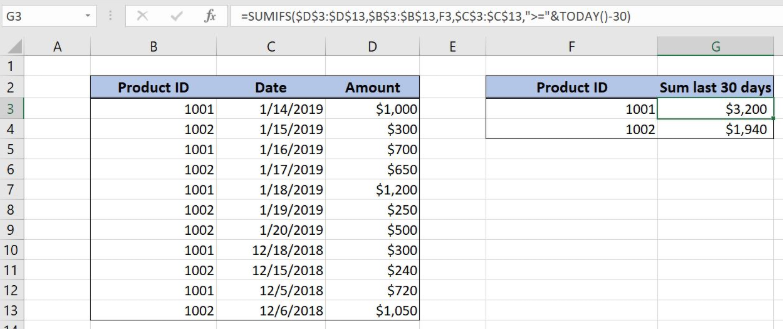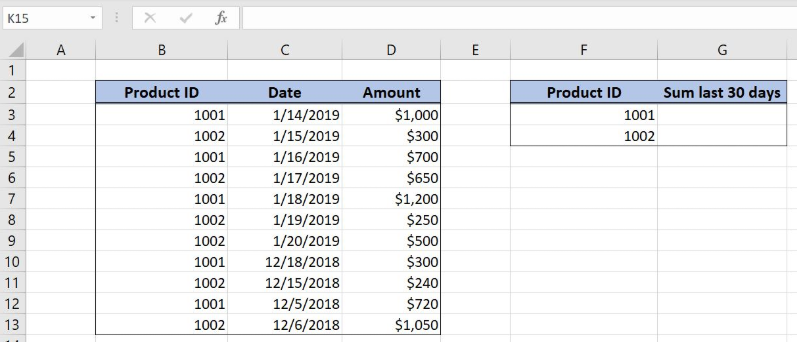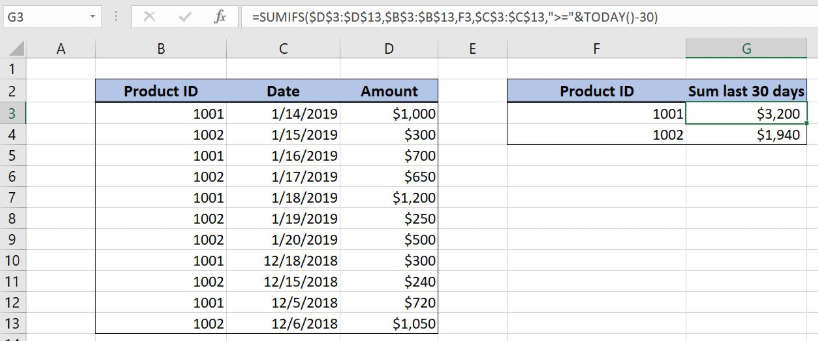Get instant live expert help with Excel or Google Sheets“My Excelchat expert helped me in less than 20 minutes, saving me what would have been 5 hours of work!”

#### Post your problem and you’ll get Expert help in seconds.

Your message must be at least 40 characters
Our professional Expert are available now. Your privacy is guaranteed.

# Sum sales in last 30 days by ID

Excel allows us to sum all values from a table with a date within the last 30 days using the SUMIFS and TODAY functions. This step by step tutorial will assist all levels of Excel users in summing sales in the last 30 days by ID.Figure 1. The final result of the formula

## Syntax of the TODAY formula

The generic formula for the TODAY function is:

`=TODAY()`

This function doesn’t have arguments and returns today date.

## Syntax of the SUMIFS formula

The generic formula for the SUMIFS function is:

`=SUMIFS(sum_range, criteria_range1, criteria1, criteria_range2, criteria2)`

The parameters of the SUMIFS function are:

• sum_range – a range with values which we want to sum
• criteria_range1 – a range where we want to set our first condition
• criteria1 – the first condition for summing the values
• criteria_range2 – a range where we want to set our second condition
• criteria2 – the second condition for summing the values

## Setting up Our Data for the Formula

The first table consists of 3 columns: “Product ID” (column B), “Date” (column C) and “Amount” (column D). The second table has 2 columns: “Product ID” (column F) and “Sum last 30 days” (column G). In column G we want to get the sum for the last 30 days for the product ID in column F.Figure 2. Data that we will use in the example

## Sum Sales in Last 30 Days by ID

In our example, we want to sum all amounts from column D if the date in column C is in the last 30 days and where the Product ID from column B is equal to F3 (1001). The result will be in the cell G3.

The formula looks like:

`=SUMIFS(\$D\$3:\$D\$13, \$B\$3:\$B\$13, F3, \$C\$3:\$C\$13, ">="&TODAY()-30)`

The sum_range is \$D\$3:\$D\$13. The criteria_range1 is \$B\$3:\$B\$13 (“Product ID” column), while the criteria1 is F3 (1001). The criteria_range2 is \$C\$3:\$C\$13 (“Date” column) and the criteria2 is “>=”&TODAY()-30. We want to get all values greater than today date minus 30 days, that’s why we need to put “>”& before TODAY()-30.

We need to fix the sum_range and both criteria_range as they are not changing when the formula is copied down the cells.

To apply the formula, we need to follow these steps:

• Select cell G3 and click on it
• Insert the formula: `=SUMIFS(D3:D9,C3:C9,G2,D3:D9,">"&G3)`
• Press enter
• Drag the formula down to the other cells in the column by clicking and dragging the little “+” icon at the bottom-right of the cell.Figure 3. Using the formula to sum sales in the last 30 days by ID

In this example, we get all the amounts which have “1001” in “Product ID” and the date is in the last 30 days. As you can see, rows 3, 5, 7 and 10 meet both conditions, so corresponding amounts are summed (\$1,000, \$700, \$1,200 and \$300). Finally, the sum in the cell G4 is \$3,200.

Most of the time, the problem you will need to solve will be more complex than a simple application of a formula or function. If you want to save hours of research and frustration, try our live Excelchat service! Our Excel Experts are available 24/7 to answer any Excel question you may have. We guarantee a connection within 30 seconds and a customized solution within 20 minutes.

### Did this post not answer your question? Get a solution from connecting with the expert.Another blog reader asked this question today on Excelchat:## Subscribe to Excelchat.coAnother blog reader asked this question today on Excelchat: